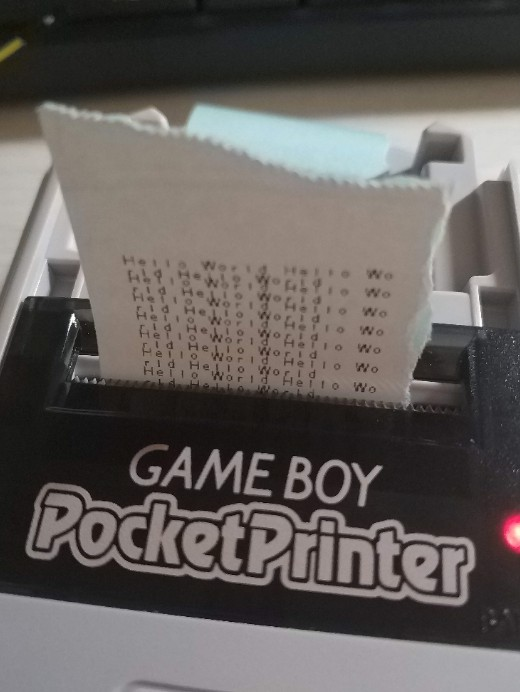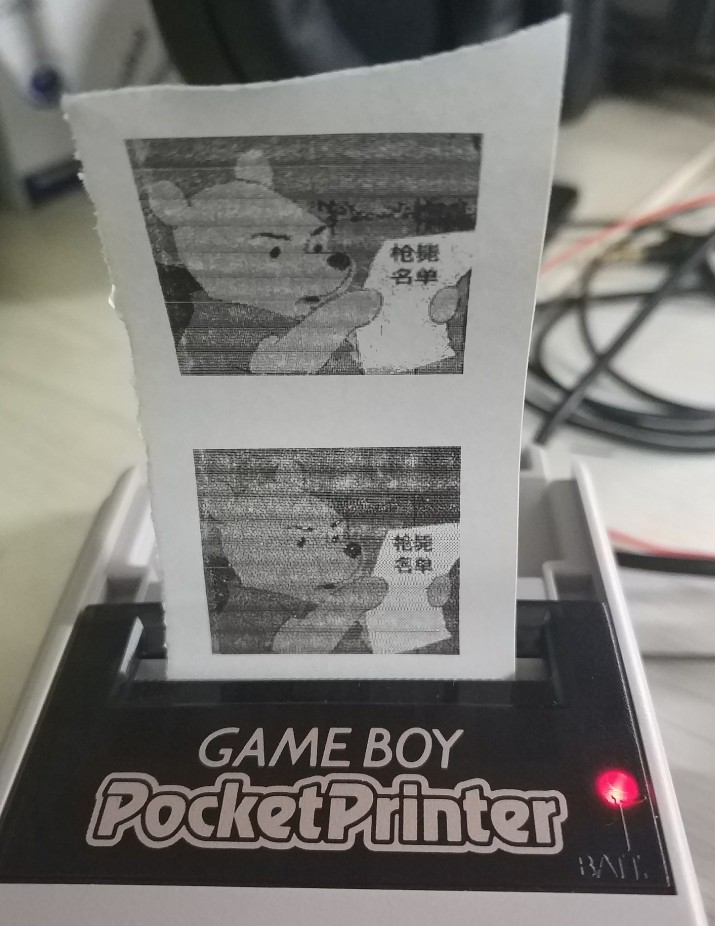## 前言

Game Boy最初是任天堂在1989年推出的8位掌机，其后又推出了GBP、GBL、GBC、GBA等衍生机型，以1.2亿台的总销量成为世界上最畅销的掌机系列。很多第三方厂商也抓住机会，针对Game Boy生产了各种神仙级别的外设（具体可参考AVGN第147集）。个人最感兴趣的是其中相机和打印机两款外设。要知道，在那个手机都不普及的90年代，一台能随时拍照还能随时打印的游戏机是多么黑科技般的存在！

## Pocket Printer 与 Pocket Camera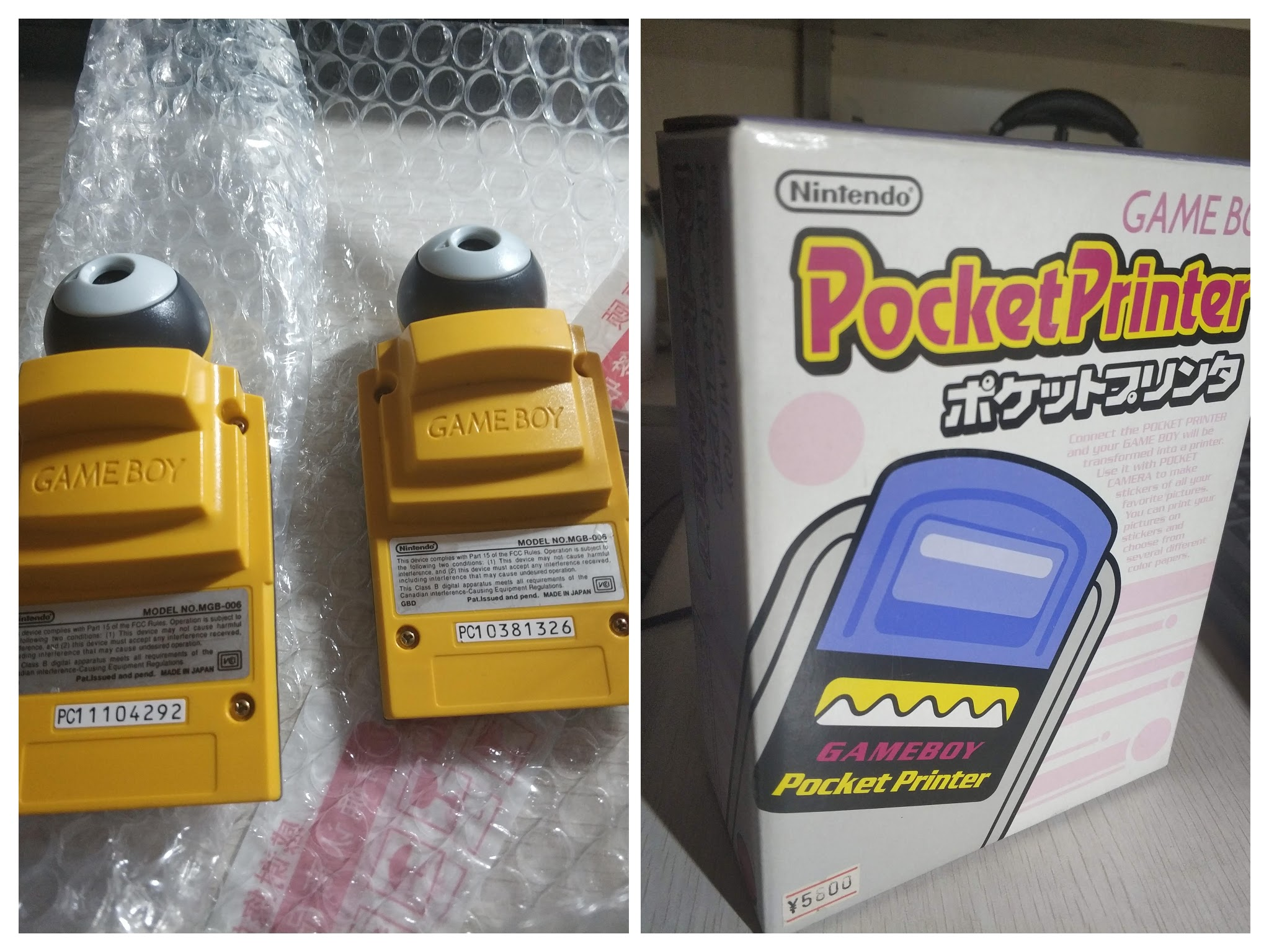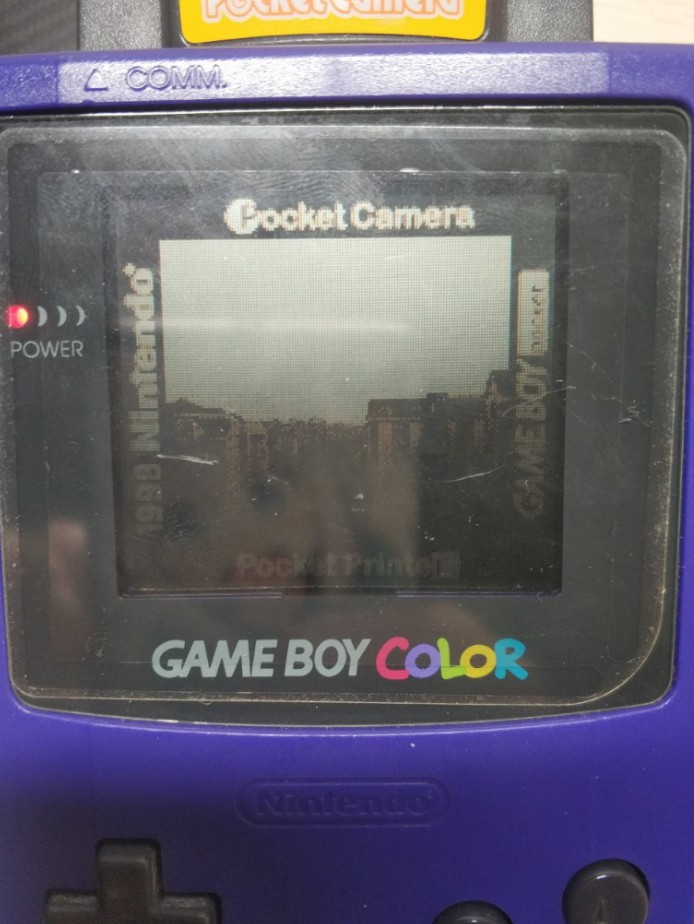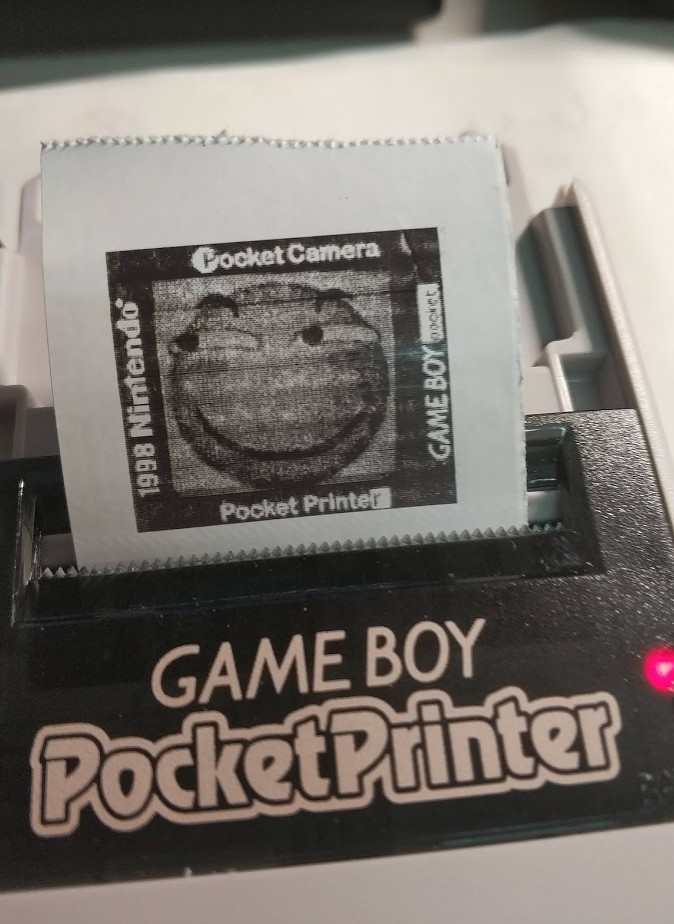## 模拟打印机接受图像

### Game Boy 通信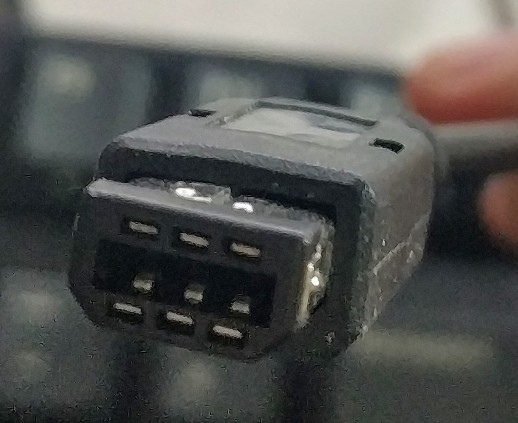`````` ___________
|  6  4  2  |
\_5__3__1_/
``````

Pin编号 功能 线缆颜色
1 VCC Orange
2 SOUT Purple
3 SIN Green
4 SD Yellow
5 SCK White
6 GND Red

（注意这里的线缆颜色是我手动测出的，不同厂家生产的规格不同，推荐自己用万用表测一遍以便备用）

Pocket Printer并没有使用到Game Boy的所有通信特性，所以本次模拟不需要使用全部的引脚，只需要2/3/5/6号就行了。

Game Boy通信的双方分为主从机两方，本次实验中GB为主机，打印机为从机。主机通过`SOUT`端口向从机发送数据，并通过`SIN`端口接受从机的响应。除此之外，主机还需要向`SCK`端口输出时钟信号，主从机通信时会共用这一个信号。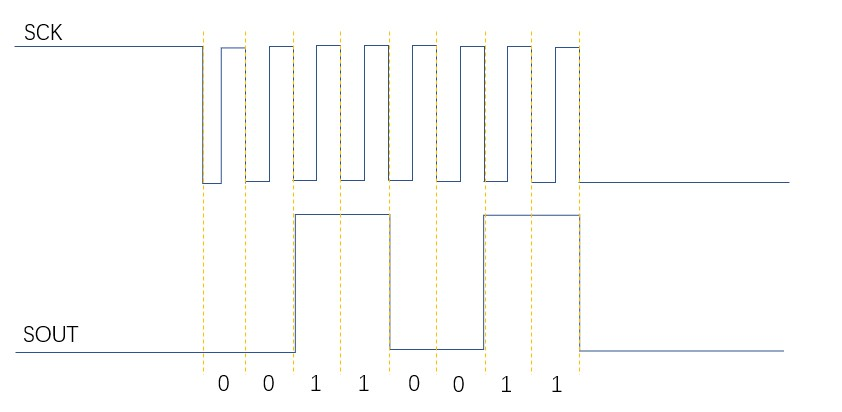`SCK`每次的下降和上升代表着一个时钟周期，从机在这个周期内读取`SOUT`的值，以此进行下去就可以得到完整的字节。这也为我们的模拟提供了大致思路：以`SCK`作为外部中断的信号，每次中断时都读取一次`SOUT`的电平。

### Pocket Printer通信协议

Byte 位置 0 1 2 3 4 5 6+X 6+X+1 6+X+2 6+X+3 6+X+4

GB发送 0x88 0x33 命令标志 压缩标志 Low Byte High Byte

• Initialize (`0x01`) 初始化指令

每次打印任务开始时发送的以一个指令，一般数据长度为0。

• Data (`0x04`) 数据传输指令

传输打印页面数据的指令。一般情况下，每次打印的页面大小与GB主机分辨率相同，而打印数据会通过包含此指令的数据包传输，每个数据包的正文大小为640字节，共9个包。所有数据传输完毕后，GB主机还会发送一个正文长度为0的空数据指令，但其用途未知。

• Print (`0x02`) 打印指令

所有打印数据传输完毕后，发送此指令开始打印。正文长度一般为4字节，第一个字节固定为`0x01`，用途未知；第二个字节定义了不同打印页面间距；第三个字节定义了打印使用的色板；第四个字节为打印色彩浓度。

• Inquiry (`0x0F`) 查询指令

此指令的预期发送时间段不固定，Game Boy主机可能会在任何时间段发送此指令查询打印机状态。可以确定的是，在打印开始后，Game Boy主机会发送一连串查询指令查询是否打印完成。

### 数据处理

``````  像素阵:                                     图像:

.33333..                     .33333.. -> 01111100 -> \$7C
22...22.                                 01111100 -> \$7C
11...11.                     22...22. -> 00000000 -> \$00
2222222. <-- 数字表示                     11000110 -> \$C6
33...33.     灰度值           11...11. -> 11000110 -> \$C6
22...22.                                 00000000 -> \$00
11...11.                     2222222. -> 00000000 -> \$00
........                                 11111110 -> \$FE
33...33. -> 11000110 -> \$C6
11000110 -> \$C6
22...22. -> 00000000 -> \$00
11000110 -> \$C6
11...11. -> 11000110 -> \$C6
00000000 -> \$00
........ -> 00000000 -> \$00
00000000 -> \$00
``````

### 硬件连线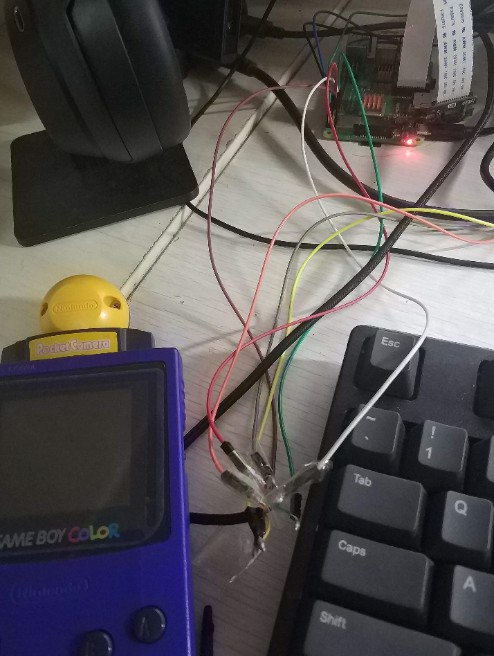Pocket Printer的通信不需要使用全部引脚，只需要连接`SIN` `SOUT` `SCK` `GND` 四个就够了。我的连接方式如下：

``````+------------+               +----------------------+
|            |               |                      |
|       SIN +------------------>GPIO.2 (BOARD #13)  |
|            |               |                      |
|       SOUT+------------------>GPIO.7 (BOARD #7)   |
|            |               |                      |
|       SCK +------------------>GPIO.0 (BOARD #11)  |
|            |               |                      |
|       GND +------------------>GND    (BOARD #9)   |
|            |               |                      |
+------------+               +----------------------+
``````

### 代码

``````#include <stdio.h>
#include <errno.h>
#include <stdlib.h>
#include <wiringPi.h>
#include <iostream>

using namespace std;

#define SCK 0
#define SOUT 7
#define SIN 2

//存放收到的Byte
unsigned char b = 0;
//前八位为ACK，后八位表示一切OK的状态，每次数据包结束时发送
int ack = {1, 0, 0, 0, 0, 0, 0, 1, 0,0,0,0,0,0,0,0};
//数据表序号
int packIndex=0;

//GameBoy packet基本信息
int dataLength = 0;
int byteIndex = 0;
int bitIndex = 0;
int checksum = 0;
bool waitForMagicByte = true;
int command;
int firstPrint=1;
int dataIndex = -1;

//Game Boy显示的行、块及坐标
int row,block,x,y=0;
//模拟Game Boy显存
string  VRAM;
//四阶灰度的代替字符
string  stringList = {" ","░","▒","▓"};

//将像素表示写入模拟显存
void toVRAM(int sign){
VRAM[row*8+y][block*16+x] = stringList[sign];
VRAM[row*8+y][block*16+x+1] = stringList[sign];
x+=2;
if(x>=16){
x=0;
y++;
if(y>=8){
y=0;
block++;
if(block>=20){
block=0;
row++;
}
}
}
}

//将模拟显存转化为字符图像输出到终端
void outputImage(){
int mark1,mark2;
int subList={128,64,32,16,8,4,2,1};
for(int i = 0; i < 9; i ++)
{
for(int j = 0; j < 640; j +=2)
{
for(int k=0;k<8;k++){
mark1=data[i][j]&subList[k];
mark2=data[i][j+1]&subList[k];
if(mark1==0&&mark2==0){
toVRAM(0);
}else if(mark1==subList[k]&&mark2==0){
toVRAM(1);
}else if(mark1==0&&mark2==subList[k]){
toVRAM(2);
}else{
toVRAM(3);
}
}
}

}
}

//处理每个字节
void handleByte(unsigned char b){

//高字节长度位
if(byteIndex==5){

if(b>0){
dataLength+=b*256;
}
return;
}

//低字节长度位
if(byteIndex==4){
if(b>0){
dataLength+=b;
}
return;
}

if(byteIndex==7+dataLength){
if(command==2&&b!=15){
ack=1;
}
}

//命令位
if(byteIndex==2){

switch (b)
{
case 1:
cout<<"Initialize "<<endl;
break;
case 4:
command=4;
dataIndex++;
cout<<"Data "<<endl;
break;
case 2:
//如果发送可打印指令，就把图像输出在命令行中
//某些情况下Game Boy初始化前会发送一个打印指令，这里手动忽略掉
if(firstPrint!=1){
outputImage();
for(int i = 1; i < 160; i ++)
{
for(int j = 0; j < 320; j ++)
{
cout<<VRAM[i][j];
}
cout << endl;
}
}else{
firstPrint=0;
}
cout<<"Print "<<endl;
break;
case 15:
cout<<"Inquiry "<<endl;
break;
default:
cout<<"Unknown "<<(int)b<<endl;
break;
}
}
//记录数据正文
if(byteIndex>=6&&byteIndex<=6+dataLength&&command==4){
data[dataIndex][byteIndex-6] = b;
return;
}
}

//终端处理函数
void myInterrupt(void){
b = b << 1;
b = b | 1;
cout<<1;
}else{
cout<<0;
}

if(waitForMagicByte){
//判断是否读到Magic Byte
if((int)b==51){
byteIndex = 2;
bitIndex = 16;
dataLength=0;
ack=0;
command=0;
waitForMagicByte=false;
cout<<"found magic byte"<<endl;
}
return;

}

//发送ACK及自身状态
if (bitIndex >= 63+dataLength*8 && bitIndex < 72+dataLength*8){
digitalWrite(2, ack[(bitIndex - (63+dataLength*8))]);
}

//是否产生了新的Byte
if((bitIndex+1)%8==0){
handleByte(b);
byteIndex++;
}
bitIndex++;

//清空计数器，准备下一个新包
if(byteIndex>=10+dataLength){
byteIndex = 0;
bitIndex = 0;
dataLength=0;
waitForMagicByte=true;
checksum = 0;
cout<<"Packet:"<<++packIndex<<endl;
}

}

int main(void){

if (wiringPiSetup() < 0)
{
fprintf(stderr, "Unable to setup wiringPi: %s\n", strerror(errno));
return 1;
}

pinMode(7, INPUT);
pinMode(0, INPUT);
digitalWrite(2, HIGH);
digitalWrite(7, HIGH);

pullUpDnControl (BUTTON_PIN, PUD_DOWN);
if (wiringPiISR(BUTTON_PIN, INT_EDGE_RISING, &myInterrupt) < 0)
{
fprintf(stderr, "Unable to setup ISR: %s\n", strerror(errno));
return 1;
}

while (1){
cout<<flush;
delay(20000);
}

return 0;
}
``````

### 最终效果## 模拟Game Boy发送打印数据

### 硬件连线

``````+------------+               +----------------------+
|            |               |                      |
|       SOUT+------------------>GPIO.2 (BOARD #13)  |
|            |               |                      |
|       SIN +------------------>GPIO.7 (BOARD #7)   |
|            |               |                      |
|       SCK +------------------>GPIO.0 (BOARD #11)  |
|            |               |                      |
|       GND +------------------>GND    (BOARD #9)   |
|            |               |                      |
+------------+               +----------------------+
``````

### 代码

``````#include <stdio.h>
#include <errno.h>
#include <stdlib.h>
#include <wiringPi.h>
#include <fstream>
#include <iostream>

using namespace std;

#define GBInitialize  0x01
#define GBData 0x04
#define GBPrint 0x02
#define GBInquiry 0x0f
#define DELAY_MS 40
#define GBClock  0
#define GBIn  7
#define GBOut  2
#define lowByte(w) ((uint8_t) ((w) & 0xff))
#define highByte(w) ((uint8_t) ((w) >> 8))

//发送一个字节
uint8_t  GBSendByte(unsigned char b){
for (uint8_t bit_pos = 0; bit_pos < 8; ++bit_pos) {
digitalWrite(GBClock, 0); // Send clock signal
if ((b << bit_pos) & 0x80) {
digitalWrite(GBOut, 1); // Write out to printer
}else {
digitalWrite(GBOut, 0);
}
delayMicroseconds (DELAY_MS);
digitalWrite(GBClock, 1);
}
delayMicroseconds (DELAY_MS);
}
}

//发送一个包
uint16_t GBSendPacket(uint8_t command, uint16_t size,uint8_t data[],int start=0) {
uint16_t status, checksum = 0x0000;
// Send magic bytes
GBSendByte(0x88);
GBSendByte(0x33);
// Send command
GBSendByte(command);
checksum += command;
// Send compression
GBSendByte(0x00);
// Send size
GBSendByte(lowByte(size));
GBSendByte(highByte(size));
checksum += lowByte(size);
checksum += highByte(size);
// Send data

uint8_t b;
for (uint16_t i = 0; i < size; ++i) {
b = data[i+start];
checksum += b;
GBSendByte(b);

}

// Send checksum
GBSendByte(lowByte(checksum));
GBSendByte(highByte(checksum));
status = GBSendByte(0x00);
status = status << 8;
status |= GBSendByte(0x00);
return status;
}

//从文件读取要发送的数据
char rawData;
std::ifstream infile("pic.bin");
infile.seekg(0, infile.end);
size_t length = infile.tellg();
infile.seekg(0, infile.beg);
memcpy( data, rawData, length);
}

int main(void)
{
if (wiringPiSetup() < 0)
{
fprintf(stderr, "Unable to setup wiringPi: %s\n", strerror(errno));
return 1;
}

pinMode(GBIn, INPUT);
pinMode(GBClock, OUTPUT);
pinMode(GBOut, OUTPUT);
pullUpDnControl(GBIn,PUD_UP);      // turn on pullup resistors
pullUpDnControl(GBOut,PUD_UP);      // turn on pullup resistors
uint8_t data={0,0,0,0,0,0,0,0};
cout<<(int)GBSendPacket(GBInitialize,0,data)<<endl;
uint8_t printData={0x01,0x00,0xE4,0x40};
uint8_t Data;
for(int i=0;i<9;i++){
cout<<(int)GBSendPacket(GBData,640,Data,i*640)<<endl;
}
cout<<(int)GBSendPacket(GBData,0,printData)<<endl;
cout<<(int)GBSendPacket(GBPrint,4,printData)<<endl;
return 0;
}
``````

### 效果展示